# Problems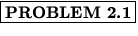The diameter of the earth, measured at the equator, is 7930 mi. Express the diameter a) in meters and b) in kilometers. Use scientific notation when expressing your answers.

Solution:

a)

 d = 7930  mi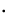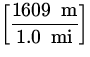= 1.28 x 107  m
b)

 d = 1.28 x 107  m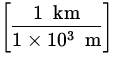= 1.28 x 104  kmThe period of a simple pendulum, defined as the time for one complete oscillation, is measured in time units and is given by:

T = 2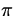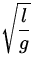where l is the length of the pendulum and g is the acceleration due to gravity, in units of length divided by time squared. Show that this equation is dimensionally consistent; that is, show that the right hand side of this equation gives units of time.

Solution: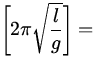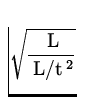== t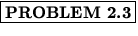A point is located in a polar co-ordinate system by the co-ordinates r = 2.5  m and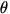= 35 o . Find the x - and y - co-ordinates of this point, assuming that the two co-ordinate systems have the same origin.

Solution: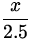= cos 35 o x = 2.5cos 35 o = 2.05  m [2mm]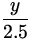= sin 35 o y = 2.5sin 35 o = 1.43  mA truck driver moves up a straight mountain highway, as shown in the figure. Elevation markers at the beginning and ending points of the trip show that he has risen vertically 0.530 km, and the mileage indicator on the truck shows that he has travelled a total distance of 3.00 km during the ascent. Find the angle of incline of the hill,.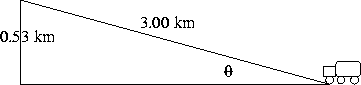Solution:

 sin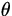== 0.177=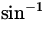(0.177) = 10.2 o